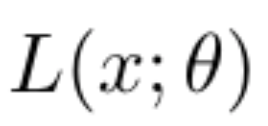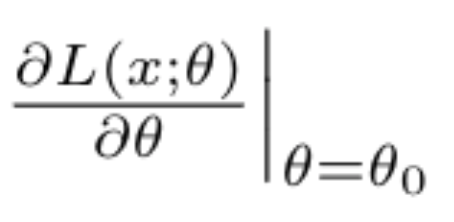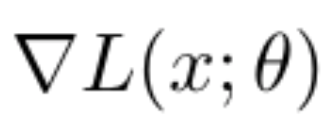# How to get the gradient of loss function w.r.t. model parameters at a particular value?

Hello,

I’m new to Pytorch, so I’m sorry if it’s a trivial question: suppose we have a loss function, and we want to get the value of, which means, get the gradient of loss function w.r.t. model parameters at a specified value.

I read a few posts of getting the gradient w.r.t. the parameters using `params.grad` method after `loss.backward()`, such as:

It gives gradient of the loss fucntion w.r.t. the current value of the model parameters, but I still couldn’t getat any specifiedI want. Could you suggest me a way to do that?

Thanks!

I think you can initialize the model parameters with the weights/values that you are interested in, and then you can compute the forward followed by backward to get the gradients.

Thank you, Vahid!

I’m interested in finding the variance ofwhen training a model across different datasets at a fixed parameter value, i.e., the variance of gradient estimator. So I think I need to stop after training the model a few epochs to calculate the gradient and do it multiple times to get the variance, can you suggest a way to do that?

Thanks

Ok, but if the you have specific parameters, and the input data \$x\$ is the same, then the gradients of these different models will also be the same, right? By training the models using different datasets the models will have different parameters, but since you want same specific parameters values, then the gradients will be the same.